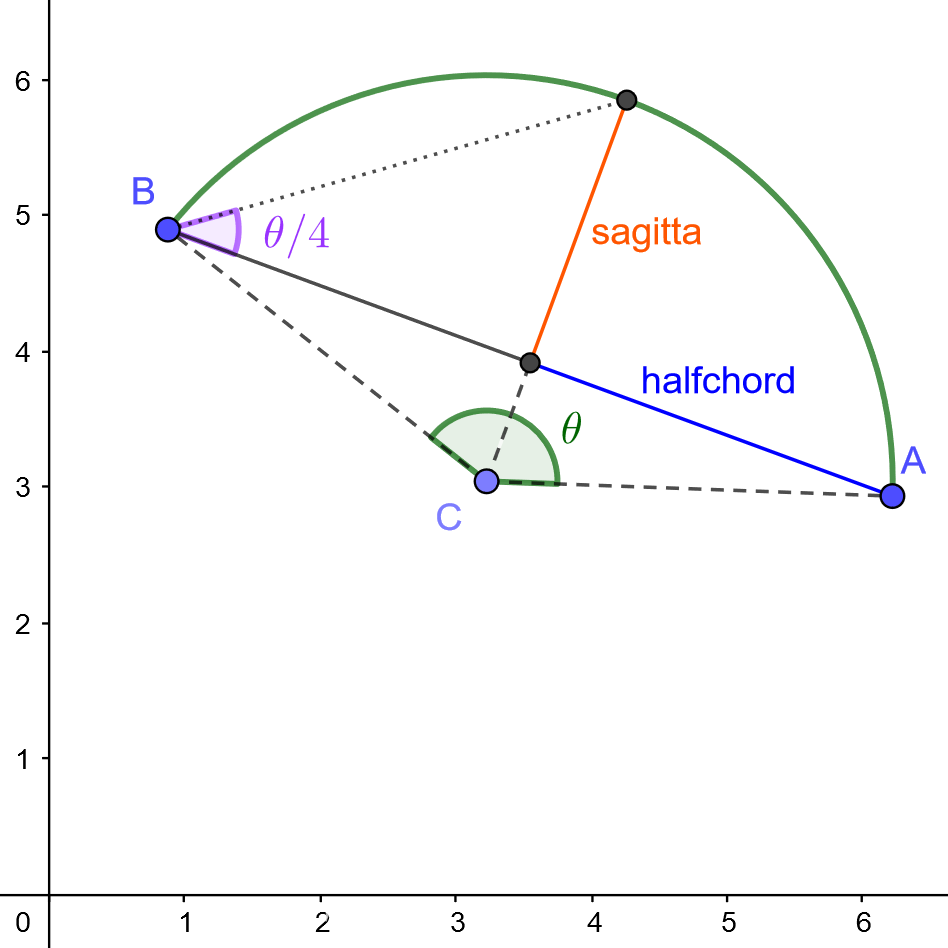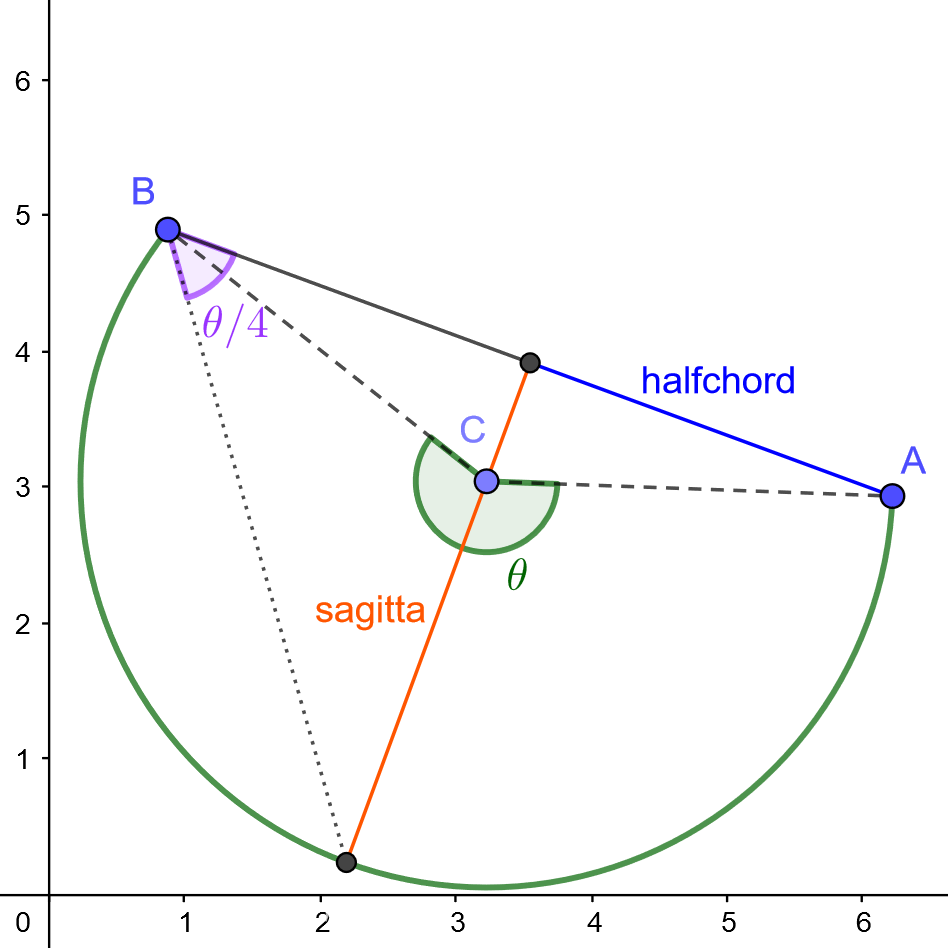Introduction

This section describes the format of nesting orders. All data are sent in a single request. The data includes parts, formats, and optimization time. The easiest way to get started is to look at the examples in Getting Started

Request

An order is sent using a POST request to https://api.nesting.almacam.com/new using the following header:

Content-Type: application/json

The Nesting Job Data Structure

Three components among four are mandatory to make a complete nesting order:

Attribute Value Description
parts array, required Description of the parts to nest
sheets array, required Description of the formats available for the nesting
time float, required The optimization time in seconds
pre_nestings array, optional Forces some nestings to be used

Parts

A part is described by its geometry, some optional properties, and its instances:

Attribute Value Description
geometry array, required The outlines of the shape
holes array, optional The holes of the shape
instances array, required The array of actual instances
protection_offset float, default = 0.0 The minimal distance with other parts
• geometry the external outlines of the part to nest as an array of contours.
• holes the holes inside the geometry, if any, described with an array of contours like for geometry.
• instances describes how many times and in which conditions this geometry can be nested: quantities, orientations allowed, ...
• protection_offset for quality reasons, it is often necessary to make sure that parts are not nested too close from each other. This defines the minimum distance between one of these part and another nested part.

Contours

A contour is a closed line that represents a geometry. There are two kinds of contours: polygonal and arc-based. In any case they shall not self-intersect.

The simplest is the polygonal contour, described with an array of vertices. One vertex is itself a plain array of its two coordinates: [x, y]. If the last vertex of the array is different from the first, they will be connected by a segment to close the polygon.

The arc-based contour is an array of elements containing information about the vertex, and the arc linking it to the next vertex. The API provides three different ways to describe an arc: with a bulge value, a sagitta length or with the center of the circle. While the latest is the most common, the two previous are prefered as they are less error prone.

The possible attributes for an arc are:

Attribute Value Description
x float, required X coordinate of the vertex
y float, required Y coordinate of the vertex
sag float, optional Sagitta of the arc
bul float, optional Bulge of the arc
cir dict("x": float, "y": float, "dir": boolean), optional Circle representing the arc
• x, y the coordinates of the current vertex, starting the arc. They also define the end point of the previous arc, if the previous element is indeed an arc.
• sag the sagitta of the arc between the current vertex and the next one. A sagitta is signed and a positive value means the arc is on the right-hand side of the chord. If 0, a segment joins both vertices. The last sagitta refers to the arc between the last vertex and the first, closing the contour. There must be no intersections. If an element contains a sag attribute, it cannot contain any other attributes than x and y.
• bul the bulge of the arc between the current vertex and the next one. A bulge is signed and a positive value means the arc is on the right-hand side of the chord. If 0, a segment joins both vertices. The last bulge refers to the arc between the last vertex and the first, closing the contour. There must be no intersections. If an element contains a bul attribute, it cannot contain any other attributes than x and y.
• cir the circle representing the arc between the current vertex and the next one. It contains the coordinates x and y of the center, and dir the direction of the arc. If dir is True, then the arc direction is counter-clockwise on the circle, and if False the arc direction is clockwise. If the circle center is not on the perpendicular bisector of the segment between the 2 vertices, it will be projected on it for further computations to ensure consistency. If an element contains a cir attribute, it cannot contain any other attributes than x and y.

If only x and y attributes are defined, a segment is used to join the next point.

Examples:

Polygonal contour of a simple square:

[
[0.0, 0.0],
[0.0, 1.0],
[1.0, 1.0],
[1.0, 0.0]
]

Arc-based contour of a simple circle defined by sagittas:

[
{
"x": 0.0,
"y": 0.0,
"sag": 0.5
},
{
"x": 1.0,
"y": 0.0,
"sag": 0.5
},
]

Arc-based contour of a simple circle defined by bulges:

[
{
"x": 0.0,
"y": 0.0,
"bul": 1.0
},
{
"x": 1.0,
"y": 0.0,
"bul": 1.0
},
]

Arc-based contour of a simple circle defined by circles:

[
{
"x": 0.0,
"y": 0.0,
"cir": {
"x": 0.5,
"y": 0.0,
"dir": True
}
},
{
"x": 1.0,
"y": 0.0,
"cir": {
"x": 0.5,
"y": 0.0,
"dir": True
}
},
]

Notions of geometry

The sagitta of an arc is the distance between the midpoint of the chord and the midpoint of the arc. A sagitta value of half the chord represents a semi-circle.

The bulge is the ratio between the sagitta and half the chord. If $\theta$ is the angle made by the arc around the circle center, then the bulge can also be seen as $tan(\theta/4)$. A bulge value of 1 represents a semi-circle.

In the figures below, there is an arc from $A(6.22, 2.94)$ to $B(0.88, 4.9)$, that has its circle center at $C(3.23, 3.04)$. The left figure represents the counter-clockwise arc of the circle, which happens to be on the right-hand side of the chord $[\overrightarrow{AB}]$. It has a sagitta of $2.06$ and a bulge of $0.73$. The right figure represents the clockwise arc of the circle, which happens to be on the left-hand side of the chord. It has a sagitta of $-3.92$ and a bulge of $-1.38$ (notice the negative sign indicating the fact that the arc is on the left-hand side of the chord).

Counter-clockwise arc Clockwise arcBoth these arcs can be defined as follows:

[
...,
{
"x": 6.22,
"y": 2.94,
ARC_INFO,
},
{
"x": 0.88,
"y": 4.9,
...
},
...
]

With ARC_INFO being one of the values below:

Type Counter-clockwise arc Clockwise arc
Sagitta "sag": 2.06 "sag": -3.92
Bulge "bul": 0.73 "bul": -1.38
Center "cir": {"x": 3.23, "y": 3.04, "dir": True} "cir": {"x": 3.23, "y": 3.04, "dir": False}

Parts Instances

Attribute Value Description
orientations array(Orientation), required The orientations allowed for this part instance
id int, required Unique identifier of the part instance
quantity int, default = 1 The quantity of this part instance
• id the unique identifier of the part, it is used later to retrieve a part in a nesting result

Orientations

Orientations are described in degrees, going counterclockwise. There are two kinds of orientations: punctual and range-based. In both cases, there is an optional flip boolean to apply a symmetry along the x axis after the rotation.

The punctual one has the following attributes:

Attribute Value Description
angle float, required the angle in [0, 359.9]
flip boolean, default = false if the part must be flipped

The range-based one defines a range of allowed orientations:

Attribute Value Description
min_angle float, required the minimum angle, [0, 359.9]
max_angle float, required the maximum angle, in [min_angle, 359.9]
flip boolean, default = false if the part must be flipped

Examples:

Free orientations without flip:

[
{
"min_angle": 0.0,
"max_angle": 359.9
}
]

Free orientations with or without flip:

[
{
"min_angle": 0.0,
"max_angle": 359.9
},
{
"min_angle": 0.0,
"max_angle": 359.9,
"flip": true
}
]

All orientations by 90 degree steps:

[
{
"angle": 0.0
},
{
"angle": 90.0
},
{
"angle": 180.0
},
{
"angle": 270.0
}
]

A single angle allowed, with or without flip:

[
{
"angle": 0.0
},
{
"angle": 0.0,
"flip": true
}
]

Sheets

Sheets represent the rectangular formats available to nest the parts.

Sheets are described using the following attributes:

Attribute Value Description
length float The length of the sheet, -1 for infinite
height float The height of the sheet
contour contour, default = None Non rectangular sheet outline, incompatible with length/height
id int, required The unique identifier of the sheet
quantity int, default = 1 The quantity of this sheet
border_gap float, default = 0.0 Avoid parts to be nested near the edge by defining a border gap
defects array(contour), default = [] The outlines of the defects
• length/height is the primary way to define a rectangular sheet.

• contour can be used instead of length/height to define non-rectangular sheets. It musts not be used for rectangular ones. The contour description is identical to the geometry descrition of parts. See section Contours for details. Please note that contour and length/height are mutually exclusive.

• defects They represent area on the sheet where no part can be nested. The protection area of a shape is allowed to intersect the defects however. The defect description is identical to the geometry descrition of parts. See the section Contours for details.

Pre-nestings

It is possible to determine in advance positions and orientations of some parts on some sheets. This is useful for instance to fill an existing nesting with more parts. Or if domain-specific constraints require a special part to be nested at a specific place.

Overlaps are not checked, parts can be placed anywhere, not necessarily fully inside the sheet. The pre-nestings informations (sheets, parts, orientations, quantities, ...) must be a valid subset of the available parts and sheets. The pre-nested quantities are substracted from the initial ones.

Pre-nestings are very similar to nesting results. Many pre-nestings can be defined. A pre-nesting contains the following attributes:

Attribute Value Description
sheet int, required the user-provided id of the sheet
nested_parts array, required the nested parts contained in that pre-nesting
quantity int, default 1 the multiplicity of this pre-nesting

And a nested-part is defined as follow:

Attribute Value Description
id int, required the user provided id of the pre-nested part
angle float, required the orientation of the pre-nested part
flip boolean, required true if the part is placed symmetrized along the x axis
position array(float), required the carthesian coordinates [x, y] of the nested part

In the following example, one of the part is placed on top right corner of the first sheet. The 9 remaining parts will then be placed automatically by the algorithm, using new sheets if necessary.

{
"parts":
[
{
"geometry": [[[0, 0], [1, 0], [1, 1], [0, 1]]],
"instances":
[
{
"id": 123,
"quantity": 10,
}
]
}
],
"sheets":
[
{
"height": 5.0,
"length": 10.0,
"id": 456,
"quantity": 4
}
],
"pre_nestings": [
{
"quantity": 1,
"sheet": 456,
"nested_parts": [
{
"position": [9.0, 4.0],
"angle": 0.0,
"id": 123,
"flip": false
}
]
}
],
"time": 3.0
}

JSON-Schema

The json-schema describing the nesting order datastructure can be found here: job_schema.js. It allows to check the validity of the jobs before sending.

Responses

201

Content-Type: application/json

{
"message": "Nesting order submitted",
"nesting_order_id": 1453303923246998305
}

400 - See Error 400 for details

Content-Type: application/json

{
"message":"An error occured",
"errors":[
{
"path":["parts", 0, "instances", 0, "orientations"],
"message":"[] is too short",
"error_code":-3000
}
]
}

401

Content-Type: application/json

{
"message": "Incorrect authentication"
}

406

Content-Type: application/json

{
"message": "This API requests json format"
}